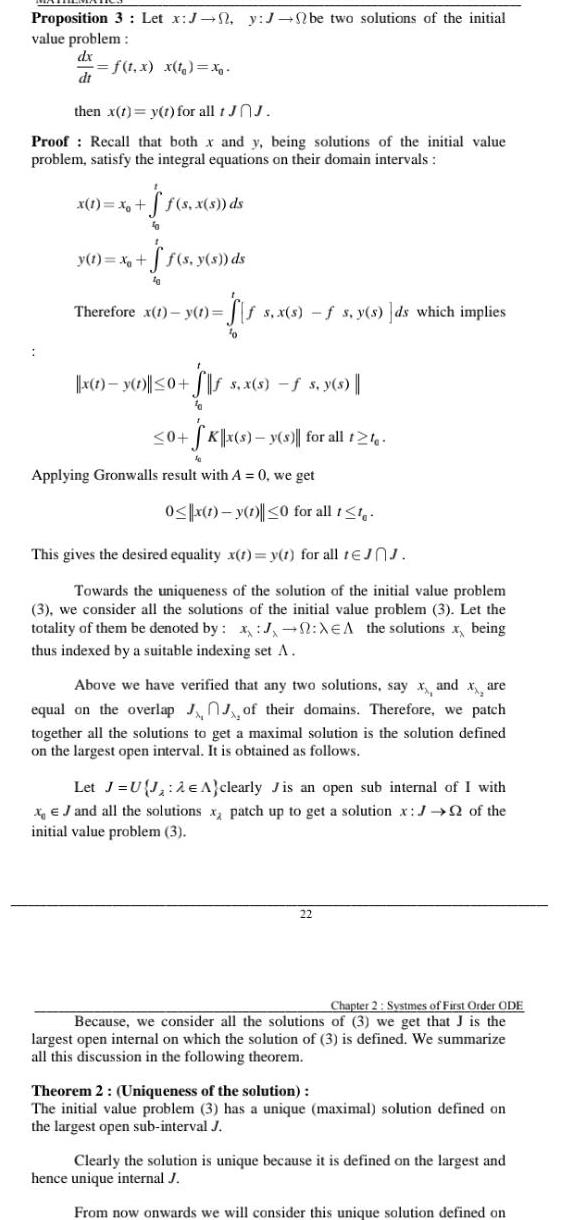Question:

# Proposition 3 Let x J y J be two solutions of the initial

Last updated: 9/18/2023Proposition 3 Let x J y J be two solutions of the initial value problem dx f t x x t x dt then x t y t for all t JOJ Proof Recall that both x and y being solutions of the initial value problem satisfy the integral equations on their domain intervals jf s x s ds fo x 1 x ff s y s ds 10 y t x Therefore x 1 y t ffs x s f s y s ds which implies Sis fo x y 0 0 S s x s f s y s 0 SK x s y s for all t2te Applying Gronwalls result with A 0 we get 0 x 1 y 1 0 for all t t This gives the desired equality x t y t for all tJJ Towards the uniqueness of the solution of the initial value problem 3 we consider all the solutions of the initial value problem 3 Let the totality of them be denoted by x J XEA the solutions x being thus indexed by a suitable indexing set A Above we have verified that any two solutions say x and x are equal on the overlap of their domains Therefore we patch together all the solutions to get a maximal solution is the solution defined on the largest open interval It is obtained as follows Let J U J 2 A clearly Jis an open sub internal of I with x J and all the solutions x patch up to get a solution x of the initial value problem 3 22 Chapter 2 Systmes of First Order ODE Because we consider all the solutions of 3 we get that J is the largest open internal on which the solution of 3 is defined We summarize all this discussion in the following theorem Theorem 2 Uniqueness of the solution The initial value problem 3 has a unique maximal solution defined on the largest open sub interval J Clearly the solution is unique because it is defined on the largest and hence unique internal J From now onwards we will consider this unique solution defined on